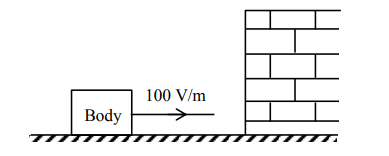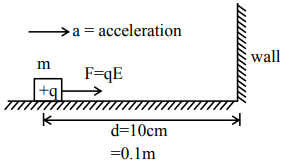# A body having specific charge

Question:

A body having specific charge $8 \mu \mathrm{C} / \mathrm{g}$ is resting on a frictionless plane at a distance $10 \mathrm{~cm}$ from the wall (as shown in the figure). It starts moving towards the wall when a uniform electric field of $100 \mathrm{~V} / \mathrm{m}$ is applied horizontally towards the wall. If the collision of the body with the wall is perfectly elastic, then the time period of the motion will be________ $\mathrm{s}$.Solution:$\mathrm{F}=\mathrm{ma}$

$\mathrm{qE}=\mathrm{ma}$

$\mathrm{a}=\frac{\mathrm{qE}}{\mathrm{m}}$

Now $\mathrm{d}=\frac{1}{2} a \mathrm{t}^{2}$

$t=\sqrt{\frac{2 d}{a}}$

$\mathrm{t}=\sqrt{\frac{2 \mathrm{~d}}{\left(\frac{\mathrm{qE}}{\mathrm{m}}\right)}}$

$t=\sqrt{\frac{2 \times 0.1}{\left(\frac{8 \times 10^{-6}}{10^{-3}}\right) \times 100}}=\frac{1}{2}$

$\therefore$ Time period $=2 \mathrm{t}=1 \mathrm{sec}$

Ans. $=1.00$